Historical Archive of the Bible Wheel Site

The Bible Wheel had been debunked by its author.
Read all about it: Debunking Myself: What A Long Strange Trip It's Been

Recent Blog Articles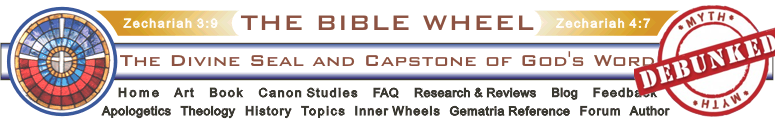Lift up your eyes on high, and behold who hath created these things, that bringeth out their host by number: he calleth them all by names by the greatness of his might, for that he is strong in power; not one faileth.

Isaiah 40:26

 Figurate Numbers Triangular Square Pentagonal Hexagonal Centered Hexagonal Star Numbers

Figurate Numbers are numbers that can be represented as a regular array of points, such as a triangle, square, or pentagon. Such numbers figure prominently in Scripture and are a highly significant part of its divine design. I am greatly indebted to Vernon Jenkins for his pioneering work in this area. His introductionto this topic and its relation to Scripture is well worth reading. Another excellent introduction - from a purely mathematical point of view - may be found in the Figurate Numberarticle on MathWorldsite.

Many of the significant numbers found in Scripture are members of geometric number sequences. For example, the entire Canon is divided into segments that contain 5, 12, or 22 Books. As discussed below, these three numbers of the second, third, and fourth terms of the Pentagonal Number Sequence. An excellent site that has a huge searchable database of such number sequences is found at The On-Line Encyclopedia of Integer SequencesTriangular Numbers: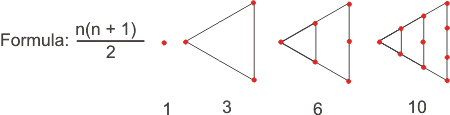The nth Triangular Number is the sum of all the numbers from 1 to n. It is represented as T(n). For example, the number of fish caught in John 21 is the seventeenth triangular number:

T(17) = 1 + 2 + ... + 16 + 17 = 153

This relation has been known and meditated upon since at least the 5th century when Augustine commented on it in his his Tractate 122on John 20:30-21:11.

Notation Change: When I first designed this website, I denoted triangular numbers with the heuristic "Sum(n)" to remind the non-mathematically trained reader that the nth triangular number is formed by summing the integers from 1 to n. But I have since come to realize that every extra symbol clutters the equations, and so have decided to change the notation to the simpler and standard "T(n)." Readers therefore may encounter triangular numbers represented either as T(n) or Sum(n) until I have completed the update.

Triangular Numbers appear frequently in Scripure. Here are a few of the more obvious, with their associated concepts:

Index Value Associated Concepts
1 T(1) = 1 All geometric numbers begin with the value "1" which is therefore called the "trivial case."
2 T(2) = 3 The Trinity: The Spirit (3) proceeds from the Father (1) and the Son (2). This manifests with great clarity on Spoke 3 as discussed on pages 164-5 of the Bible Wheel book, reproduced online here.
3 T(3) = 6 Creation of Man and Cosmos: Six = 600 = Cosmos. Six is a "perfect number" in that 1 + 2 + 3 = 1 x 2 x 3 = 6. It is profoundly integrated with the Creation Holograph as well as Spoke 6 of the Bible Wheel.
4 T(4) = 10 Number of the Ten Commandments and base of the Bibical numerical system. It is profoundly integrated with Spoke 10 of the Bible Wheel.
7 T(7) = 28 The Number of words (7) and letters (28) of the Creation Holograph (Genesis 1.1) which sums to 2701 = T(73)! [See below]
11 T(11) = 66 The Number of Books in the Wheel, and the value of the word Wheel itself:
 Wheel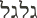Galgal = 66
19 T(19) = 190 The Index and the Value are related through this fundamental identity from Genesis 3:
 RibTsalo = 190 = T(19) = T(Eve)
23 T(23) = 276 The Number of souls saved in Paul's shipwreck (Acts 27.37)
24 T(24) = 300 The Value of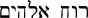(Ruach Elohim, The Spirit of God). This is an extremely profound relationship.
32 T(32) = 528 The KEY to the Bible = T(32)! (Note: The Hebrew for Heart is Lev = 32)
36 T(36) = 666 The Number of the Beast (Rev. 13.18).
43 T(43) = 946 The Spirit = T(43) (Note: The Hebrew word for "Greatness" is Gadol = 43. This is the primary role of Holy Spirit - to magnify the Father and the Son.
73 T(73) = 2701 The sum of Genesis 1.1, the Creation Holograph. It is expressible as a very simple combinations of elements from the Holographic Generating Set: 2701 = 0 = T(C) = T(B) + 0
112 T(112) = 6328 The sum of Genesis 1.1 + John 1:1 from the Creation HyperHolograph. The index 112 = YHVH Elohim (The LORD GOD) from the Unity Holograph (Shema). It also is expressible as a simple combination of the Holographic Generating Set: 6328 = 0 + C2

Square Numbers:Square Numbers are associated with structure - e.g. the Altar, The Temple, and the New Jerusalem are all described as foursquare, as was the camp that formed a Cross in the Wilderness. This is particularly astouding in light of this pair of identities:

 The New JerusalemHeh Kaine Ierusalem
= 961 = 31 x 31 =
 Heaven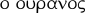Ho Ouranos

We also have the identity:

 The Kingdom of God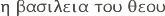Heh Basileia tou Theou = 1521 = 39 x 39

The Kingdom of God is ruled by Truth, which is a Square Number in both Hebrew and Greek:

HebrewGreek
 Truth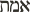Emet = 441 = 21 x 21
 Truth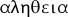Aletheia = 64 = 8 x 8

This manifests in popular numerical slang that takes "foursquare" as representing Truth, Honesty, and Integrity.

Another significant Square is found in the Number 144, which represents the Elect of God and eternal citizens of the New Jerusalem:

 The ElectHay Ekloge = 144 = 12 x 12

This is tremendously significant, in that the pattern based on the Number 12 was established in the Hebrew Testament, yet it manifests in the intrinsic alphanumeric structure of the Greek language and the explicit message of the text of the New Testatment (Revelation 14.1)!

Pentagonal Numbers:

Pentangular Numbers are extremely significant in the structure of the Christian Canon in that the entire structure is built upon the first three:The Number 5 integrates with the Creation of Life on the Fifth Day (which also records the first Blessing) and the Fifth Commandment which is "the first commandment with promise."

Here are a few of the more significant Pentagonal Numbers and their associated concepts:

Index Value Associated Concepts
2 5 The Number of Books in four canonical divisions: Torah, Major Prophets, Wisdom, and NT History.
3 12 The Number of Books in two canonical divisions: OT HIstory and Minor Prophets. This Number relates to the Twelfth Letter Lamedh, which signifies an ox-goad and symbolizes the idea of To Teach. Hence Lamed is the root of Talmud, and Jesus had 12 disciples, and so forth.
4 22 The Number of Letters in the Hebrew Alphabet, the Number of Spokes of the Wheel and the Number of Books in one canonical division: NT Epistles. The Number 22 is the root of the KEY (528 - Triangular, see above) given in Isaiah 22.22.
9 117 Scripture is divided into categories based on Pentangular Numbers 5, 12, and 22. This theme is continued in Psalm 117, which is the dividing chapter of the Bible (i.e. it is chapter 595 at the center of 1189 chapters, as discussed by Mark Darroch in his article Dividing the Waters of the Word found in the Collaboration section. Darroch then links the structure of Scripture to this identity from Genesis 1.6:
 And let it divideVeyhi MavDeel = 117
This further integrates with the Word of the Lord through the identity:
 Saith the LordNa'am YHVH = 117
This phrase occurs in 267 verses of the Old Testament, 167 of which appear in the Book of Jeremiah on Spoke 2. This integrates with the concepts of the Division and the Word which dominate that Spoke.
14 287 This refers to the Wonderful Counselor, the Spirit of Wisdom who is My Help. It integrates beautifully with the general concepts of Scripture subsumed in the Pentagonal Number Category.
19 532 This is the value of Alpha - the symbol of Almighty God - and many of His Titles, such as "The Holy Lord God."
27 1080 This is the value of "The Holy Spirit". This integrates with its index 27 = 3 x 3 x 3 and the Comforter = 810 = 3 x 3 x 3 x 30, and all of this relates to the Third Person of the Holy Trinity. Glory! Praise God!

Hexagonal Numbers:Hexagonal Numbers are a subsequence of Triangular Numbers, generated by the Triangular Numbers with odd indices.

Index Value Associated Concepts
2 6 The Number Six is the Number of Man, Work, and Cosmos. Its relation to these concepts arises from the creation of Man and the completion of God's Work on the Sixth Day. It also integrates with the Six Directions of the Cosmos is amplified in this pair of identites:
 WorldCosmos
= 600 =
 SixShaysh
4 45 The value of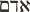(Adam). Using the Sofit Value of the final letter Mayim yields Adam = 610 =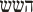(HaShaysh, The Six).
7 66 The Seventh Hexagonal Number coincides with the number of books in the Canon, while the Index coincides with the number of Canonical Divisions. This all coheres with God's use of the Number 6 in Creation.

Centered Hexagonal Numbers:These Numbers, along with the Star Numbers (see below) are essential to the holographic structure of Holy Scripture.

 Index Value Associated Concepts 2 7 This is the premier number in all of Scripture, as discussed in some detail in the article Seven and Scripture. 3 19 The Number 19 is the number of Physical Manifestation. It appears reiteratively in the Grace Manifest Holograph in eight nested word clusters. 4 37 The Number 37 is the only number less that 1261 that is both a Centered Hexagonal and a Star Number. It is the Hexagonal core of the fourth Star Number 73, and this palindromic Hexagon/Star pair forms two of the elements of the Holographic Generating Set (27, 37, 73) found throughout the components of the Creation HyperHolograph.

Star Numbers: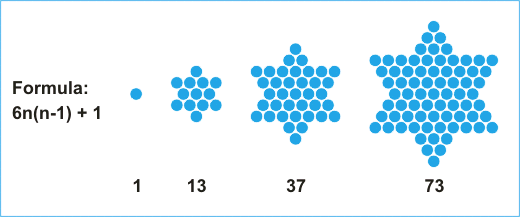These four numbers figure prominently in many holographs. They are deeply integrated with the Lord's Work of Creation. Their beauty knows no limit. What glory in the Lord's Work! How great is His understanding!

Index Value Associated Concepts
2 13 This Number is the basis of the Unity Holograph which is built upon four nested word clusters that sum to multiples of the Number (= One = Love). This further manifests in 1 Corinthians 13 - the Love Chapter.
3 37 The Numbers 37 and 73 (the fourth Star Number) are palindromic primes. They are both a kind of "division of unity" into two primes 10 = 3 (Trinity) + 7 (Fullness). Their product is the sum of the Creation Holograph: 2701 = 37 x 73 = T(73). The Number 37 is the Ordinal Value of the word Wisdom:
 Wisdom (Ord)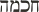Chokmah = 37
4 73 The Numbers 37 (the third Star Number) and 73 are palindromic primes. Their product is the sum of the Creation Holograph (see above) and the Number 73 is one of the fundamental primes in the Logos Holograph. The primary meaning of the Number 73 is found in this fundamental identity:
 Wisdom (Std)Chokmah = 73

This means that both the Standard and the Ordinal Values of Wisdom are Centerer Hexagonal Numbers, with their proiduct giving the value of the Creation Holograph, the sum of Genesis 1.1! This certainly adds an unexpected dimension to the teaching that (Jeremiah 10.12):

He hath made the earth by his power, he hath established the world by his wisdom, and hath stretched out the heavens by his discretion.

10 541 The value of Israel, The Commandments, and Stone Tablets. Simply one of the most astounding discoveries! How is it that the Israel chose this, the Tenth Star of David, as its national symbol? Is it conceiveable that this is a "mere coincidence?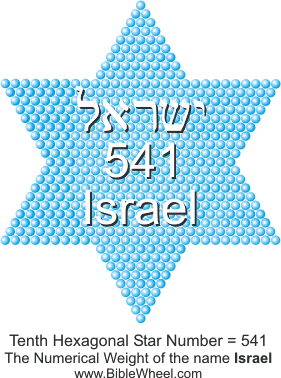Hexagon / Star Pairs:

Centered Hexagonal and Star Numbers all come in pairs. This is extremely significant because the Creation Holograph and Logos Star are built off such pairs.The product of any such pair is always a Triangular Number because Star(n) + 1 is always twice the value of its Hexagon pair: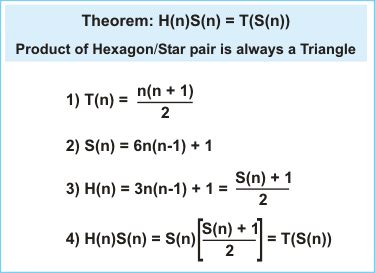Some examples:

• T(S(2)) = T(13) =    91 =   7 x 13 = H(2)S(2)
• T(S(3)) = T(37) =  703 = 19 x 37 = H(3)S(3)
• T(S(4)) = T(73) = 2701 = 37 x 73 = H(4)S(4)

These numbers play an essential role in the Creation Holograph, the alphanumeric structure of Genesis 1:1.